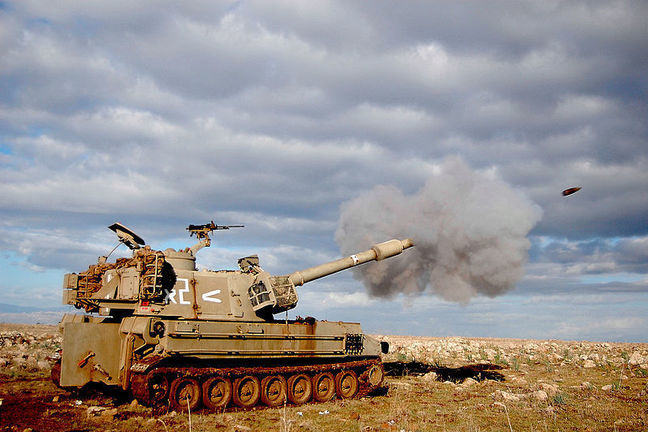## Want to keep learning?

This content is taken from the UNSW Sydney's online course, Maths for Humans: Linear, Quadratic & Inverse Relations. Join the course to learn more.
2.14

## UNSW SydneyA trajectory is born

# A Cannonball's Trajectory

How does a cannonball or shell fly? What kind of trajectory does it make? If we ignore air resistance and the curvature of the earth the answer is that the trajectory is parabolic. This classical curve is an excellent first approximation to understanding the motion of a wide range of projectiles, from boulders tossed by catapults to baseballs.

In this step we will see that the parabola makes its appearance again in projectile motion.

## Paths of projectiles

Humans have been throwing rocks, spears and boomerangs at their enemies, their prey, and occasionally their kin, for no doubt tens or even hundreds of thousands of years. Early human hunters would have had an accurate qualitative understanding of how high to aim a projectile to make it achieve a certain range.

Later, with the advent of ancient siege weapons, such as the catapult or trebuchet, this ability to throw something heavy a long distance, or over high walls, allowed a decisive advantage.© “Trebuchet at Château des Baux, France (reconstruction)” ChrisO/Wikimedia Commons CC BY SA 3.0

But what paths do such objects actually make as they fly through the air? Aristotle theorized that projectiles were pushed along by an external force which was transmitted through the air. In medieval times, this “impetus” was thought to be responsible for propelling the particle along till it was expended, at which point the object fell more or less straight to the ground.

## A parabolic view

A correct mathematical analysis of the actual path of a projectile had to wait for European developments in geometry and physics. Galileo had the key insight that the vertical and horizontal components of motion were actually independent, and that trajectories formed continuous smooth curves without abrupt edges.

With Newton’s laws of motion and law of Gravitation, it was discovered that the path of an ideal trajectory (ignoring air resistance and the curvature of the earth) was parabolic.

This is now a different kind of parabola from the one we discussed with Galileo’s ball. Here we are discussing the trajectory of, say, a cannonball which is shot from some angle into the sky, as it traverses both horizontal distance $$\normalsize{(x)}$$, and at the same time goes up and then down in the vertical direction $$\normalsize{(y)}$$ as shown in this figure.So the image of the cannonball may be traced as a curve in the $$\normalsize{x-y}$$ plane, with $$\normalsize{y}$$ playing the role of height. The curve is an approximation to what you would actually see if you were able to watch the entire trajectory from some distance away. We may suppose that the cannon is fired from the point $$\normalsize{x=0}$$ at a height $$\normalsize{y=c}$$.

The basic quadratic relation is then $$\normalsize{y=-ax^2+bx+c}$$. Again the $$\normalsize{bx}$$ term is the result of the initial velocity and its angle, while the $$\normalsize{-ax^2}$$ is the contribution made by gravity to bring the cannonball back down to earth.

Q1 (E): What would happen if there was no gravity?

Q2 (E): One of the pleasant consequences of knowing that a trajectory is parabolic is that we can use what we know about the geometry of a parabola to say something about the trajectory. For example a cannonball is shot down an abandoned road from ground level and falls to ground $$\normalsize{3}$$ kilometers away. Where did it reach its maximum?

Q3 (M): This analysis is all very well, but it certainly does not apply to a boomerang. Why not?

## Aiming for maximum range

A key question for artillery is at what inclination to fire a cannon or gun to maximize range. With the understanding of the quadratic nature of the trajectory, this can be determined: it is a slope of $$\normalsize{1}$$, or angle of $$\normalsize{45}$$ degrees.

A1. If there is no gravity, then the coefficient of $$\normalsize{a}$$ in $$\normalsize{y=-ax^2+bx+c}$$ would be zero, giving $$\normalsize{y=bx+c}$$. The particle would just shoot off into space exactly in the direction you fired it, at a constant speed.
A2. We know that the maximum of a parabolic function occurs exactly half-way between its zeros. In this case the zeros are where the cannonball is at ground level, namely the place of firing and the place where it lands. So the maximum must occur half way: $$\normalsize{3/2}$$ of a kilometer down the road.